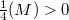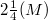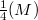﻿

### On the Gauss map of embedded minimal tubes

#### Abstract

The Gaussian image of the minimal tubes of arbitrary dimension is studied. If the angle between the flow-vector of such a surface M and its axe is equal to$𝛼(M) > 0$ then the diameter of the Gauss image of M is at least$2𝛼(M)$. As a consequence we show that the length of a two dimensional minimal tube M can be estimated by the angle$𝛼(M)$ and the total Gaussian curvature of M.

DOI Code: 10.1285/i15900932v19n1p7

Keywords: Minimal tubes; Gaussian map; Total gaussian curvature; Flow vector

Classification: 53A10; 53A55

Full Text: PDF